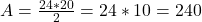## The base and altitude of a triangle are 24 m and 20 m respectively. Then the area of the triangle is _____

Question

The base and altitude of a triangle are 24 m and 20 m respectively. Then the area of the triangle is _____

in progress 0
3 months 2021-08-25T17:19:42+00:00 1 Answers 1 views 0

Then the area of the triangle is 240 m²

Step-by-step explanation:

Area of a triangle:

The area of a triangle is given by the multiplication of its height and altitude, divided by 2.

In this question:

Base = 24m, altitude = 20m. SoThen the area of the triangle is 240 m²